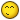Script Needed47

Hi Guys,

I need help, is there something like this already?

I need a script or something similar. I don't know much about coding in Metatrader.

Here is an example.

I want a script to place my buy or sell order, I decide at what rate. Lets say I buy EURUSD at 1.1000. And I want to place 3 lots. Spread is 4 pips for example. When my target is reached at 1.1012 then I want to sell 2 lots and set the 3rd lot to break even. The target is equal to the spread multiplied by the number of lots (in this case 3).

I need the amount of lots to be configurable. The target should be calculated on the amount of lots multiplied by the spread. So for a buy the target is 1.1012, and for a sell it would be 1.0988. Break even is calculated.

What would you suggest suggest? Is this possible?

I hope someone can help me out.

Regards

Brendan